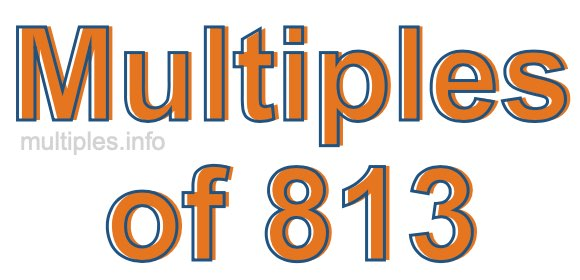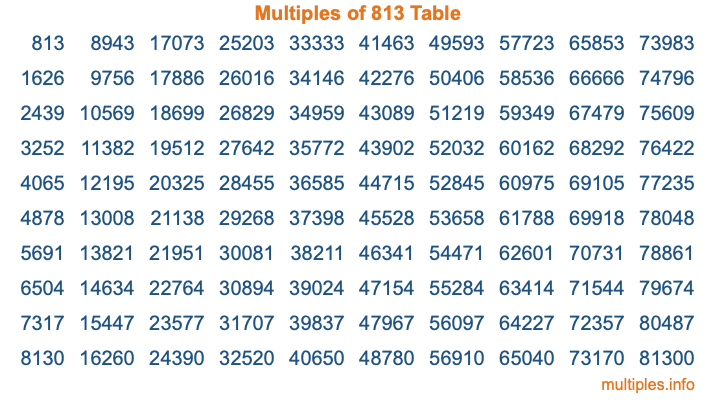Multiples of 813Welcome to the Multiples of 813 page. Here we will first teach you everything you will ever need to know about the multiples of 813, and then give you a study guide summary of everything we taught you to make sure you remember it all. Use this page to look up facts and learn information about the multiples of 813. This page will make you a multiples of eight hundred thirteen expert!

Definition of Multiples of 813
Multiples of 813 are all the numbers that when divided by 813 equal an integer. Each of the multiples of 813 are called a multiple. A multiple of 813 is created by multiplying 813 by an integer.

Therefore, to create a list of multiples of 813, you start with 1 multiplied by 813, then 2 multiplied by 813, then 3 multiplied by 813, and so on for as long as you want. Thus, the list of the first five multiples of 813 is 813, 1626, 2439, 3252, and 4065. To see a larger list of multiples of 813, see the printable image of Multiples of 813 further down on this page. We also have a category where you can choose any nth multiple of 813.

Multiples of 813 Checker
The Multiples of 813 Checker below checks to see if any number of your choice is a multiple of 813. In other words, it checks to see if there is any number (integer) that when multiplied by 813 will equal your number. To do that, we divide your number by 813. If the the quotient is an integer, then your number is a multiple of 813.

Is  a multiple of 813?

Least Common Multiple of 813 and ...
A Least Common Multiple (LCM) is the lowest multiple that two or more numbers have in common. This is also called the smallest common multiple or lowest common multiple and is useful to know when you are adding our subtracting fractions. Enter one or more numbers below (813 is already entered) to find the LCM.

Check out our LCM Calculator if you need more details about the Least Common Multiple or if you need the LCM for different numbers for adding and subtraction fractions.

nth Multiple of 813
As we stated above, 813 is the first multiple of 813, 1626 is the second multiple of 813, 2439 is the third multiple of 813, and so on. Enter a number below to find the nth multiple of 813.

th multiple of 813

Multiples of 813 vs Factors of 813
813 is a multiple of 813 and a factor of 813, but that is where the similarities end. All postive multiples of 813 are 813 or greater than 813. All positive factors of 813 are 813 or less than 813.

Below is the beginning list of multiples of 813 and the factors of 813 so you can compare:

Multiples of 813: 813, 1626, 2439, 3252, 4065, etc.

Factors of 813: 1, 3, 271, 813

As you can see, the multiples of 813 are all the numbers that you can divide by 813 to get a whole number. The factors of 813, on the other hand, are all the whole numbers that you can multiply by another whole number to get 813.

It's also interesting to note that if a number (x) is a factor of 813, then 813 will also be a multiple of that number (x).

Multiples of 813 vs Divisors of 813
The divisors of 813 are all the integers that 813 can be divided by evenly. Below is a list of the divisors of 813.

Divisors of 813: 1, 3, 271, 813

The interesting thing to note here is that if you take any multiple of 813 and divide it by a divisor of 813, you will see that the quotient is an integer.

Multiples of 813 Table
Below is an image of the first 100 multiples of 813 in a table. The table is in chronological order, column by column. The first column has the first ten multiples of 813, the second column has the next ten multiples of 813, and so on.The Multiples of 813 Table is also referred to as the 813 Times Table or Times Table of 813. You are welcome to print out our table for your studies.

Negative Multiples of 813
Although not often discussed or needed in math, it is worth mentioning that you can make a list of negative multiples of 813 by multiplying 813 by -1, then by -2, then by -3, and so on, to get the following list of negative multiples of 813:

-813, -1626, -2439, -3252, -4065, etc.

Multiples of 813 Summary
Below is a summary of important Multiples of 813 facts that we have discussed on this page. To retain the knowledge on this page, we recommend that you read through the summary and explain to yourself or a study partner why they hold true.

There are an infinite number of multiples of 813.

A multiple of 813 divided by 813 will equal a whole number.

813 divided by a factor of 813 equals a divisor of 813.

The nth multiple of 813 is n times 813.

The largest factor of 813 is equal to the first positive multiple of 813.

813 is a multiple of every factor of 813.

813 is a multiple of 813.

A multiple of 813 divided by a divisor of 813 equals an integer.

813 divided by a divisor of 813 equals a factor of 813.

Any integer times 813 will equal a multiple of 813.

Multiples of a Number
Here you can get the multiples of another number, all with the same attention to detail as we did for multiples of 813 on this page.

Multiples of
Multiples of 814
Did you find our page about multiples of eight hundred thirteen educational? Do you want more knowledge? Check out the multiples of the next number on our list!

Copyright  |   Privacy Policy  |   Disclaimer  |   Contact